# 数字电路基础知识

## 数制与码制

$$(526)_8=5*8^2+2*8^1+6*8^0=(342)_{10}$$

$$(2A.7F)_H=2*16^1+10*16^0+7*16^{-1}+15*16^{-2}=(42.4960937)_D$$

$$D=\sum K_iN^i$$

### 二进制的补码

• 最⾼高位为符号位（0 为正，1 为负）
• 正数的补码和它的原码相同
• 负数的补码 = 数值位逐位求反 + 1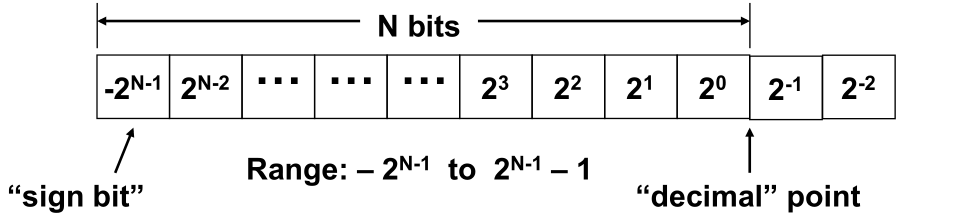+5 = （0 0101）
-5 = （1 1011）

1101.0110
= –2 3 + 2 2 + 2 0 + 2 -2 + 2 -3
= – 8 + 4 + 1 + 0.25 + 0.125
= – 2.625

### 码制

• 4 位 BCD 码
• ASCII
• 格雷码：编码顺序依次变化，按表中顺序变化时，相邻代码只有⼀一位改变状态

## 逻辑代数基础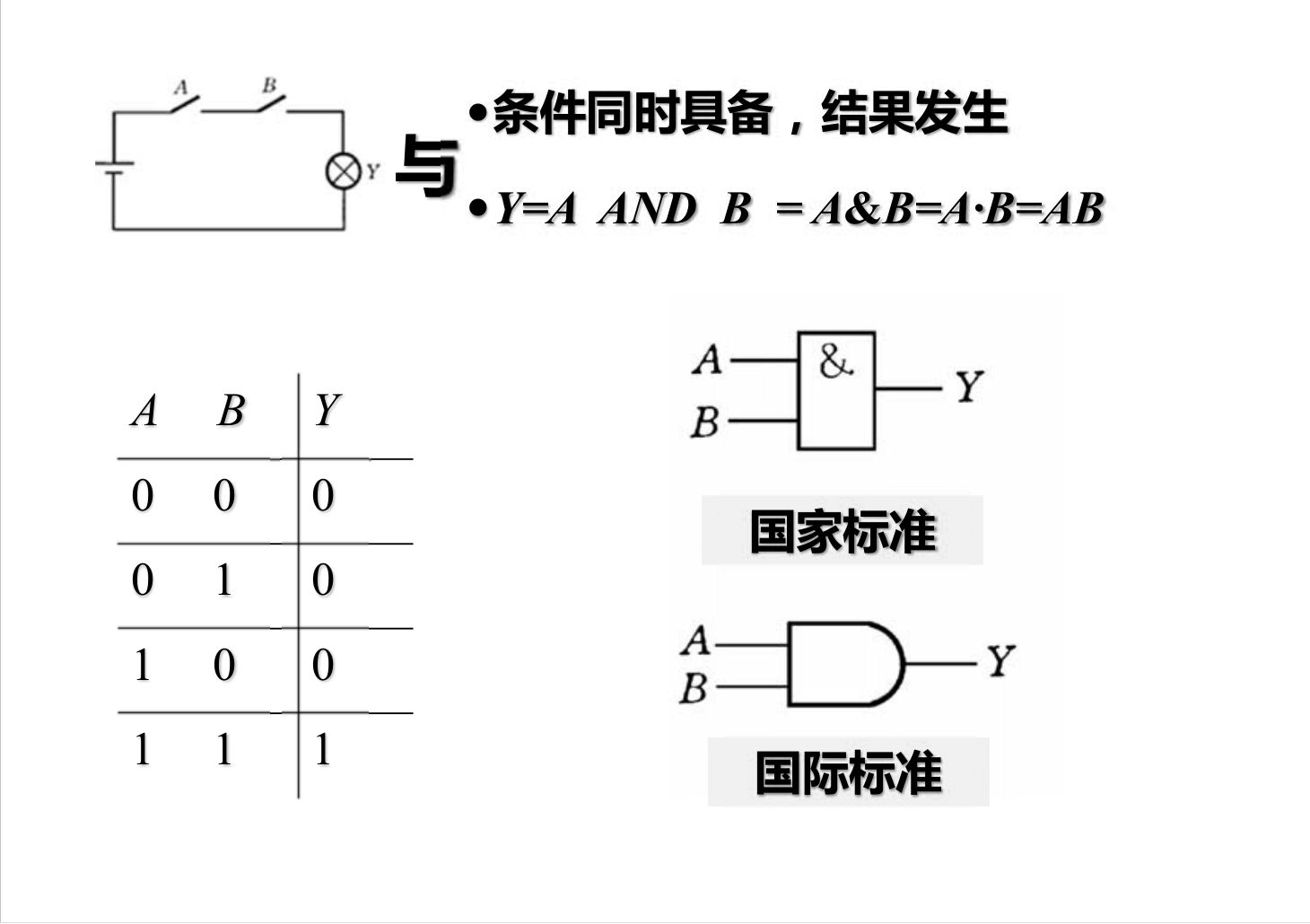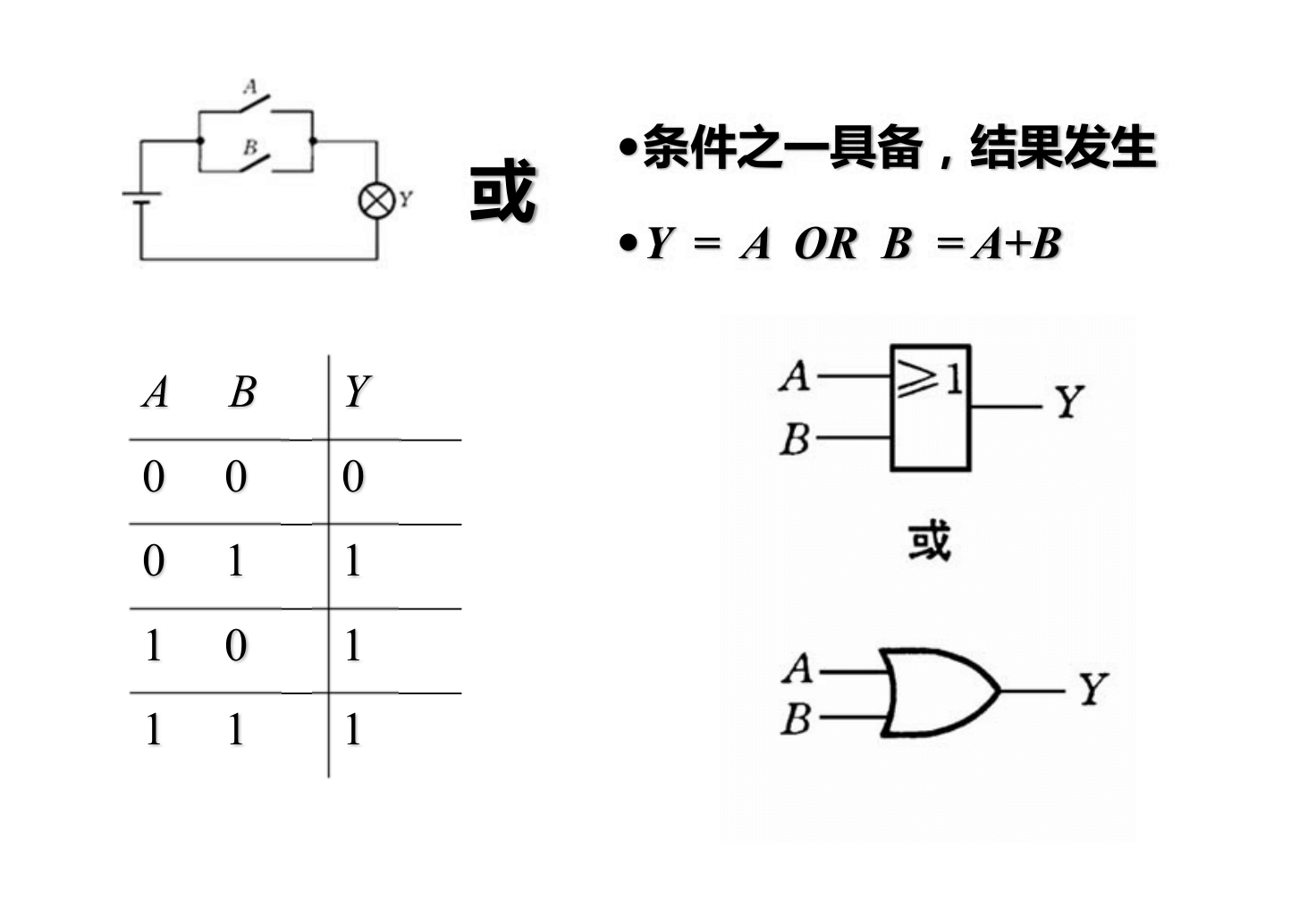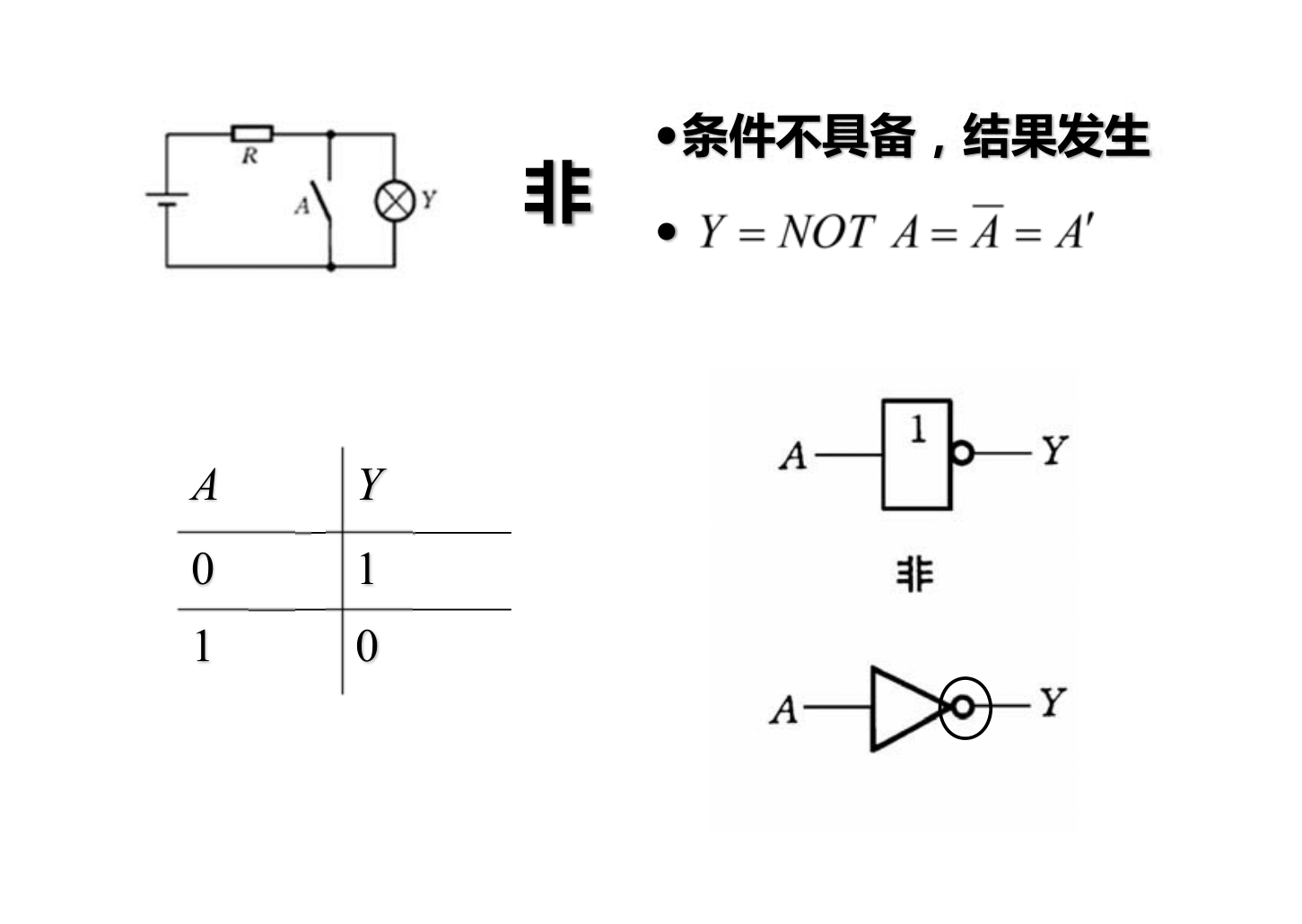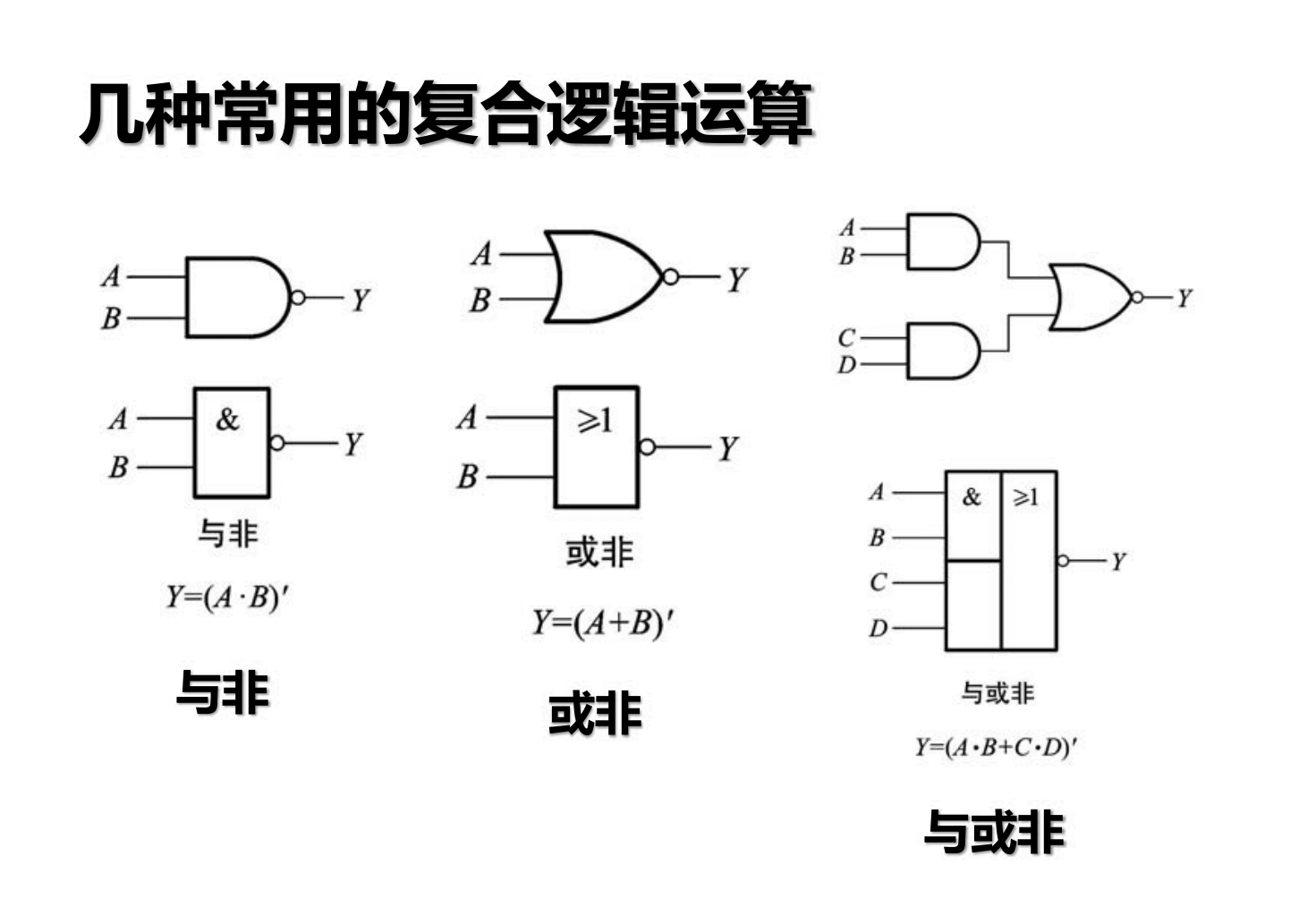$$Y=A'B+AB'$$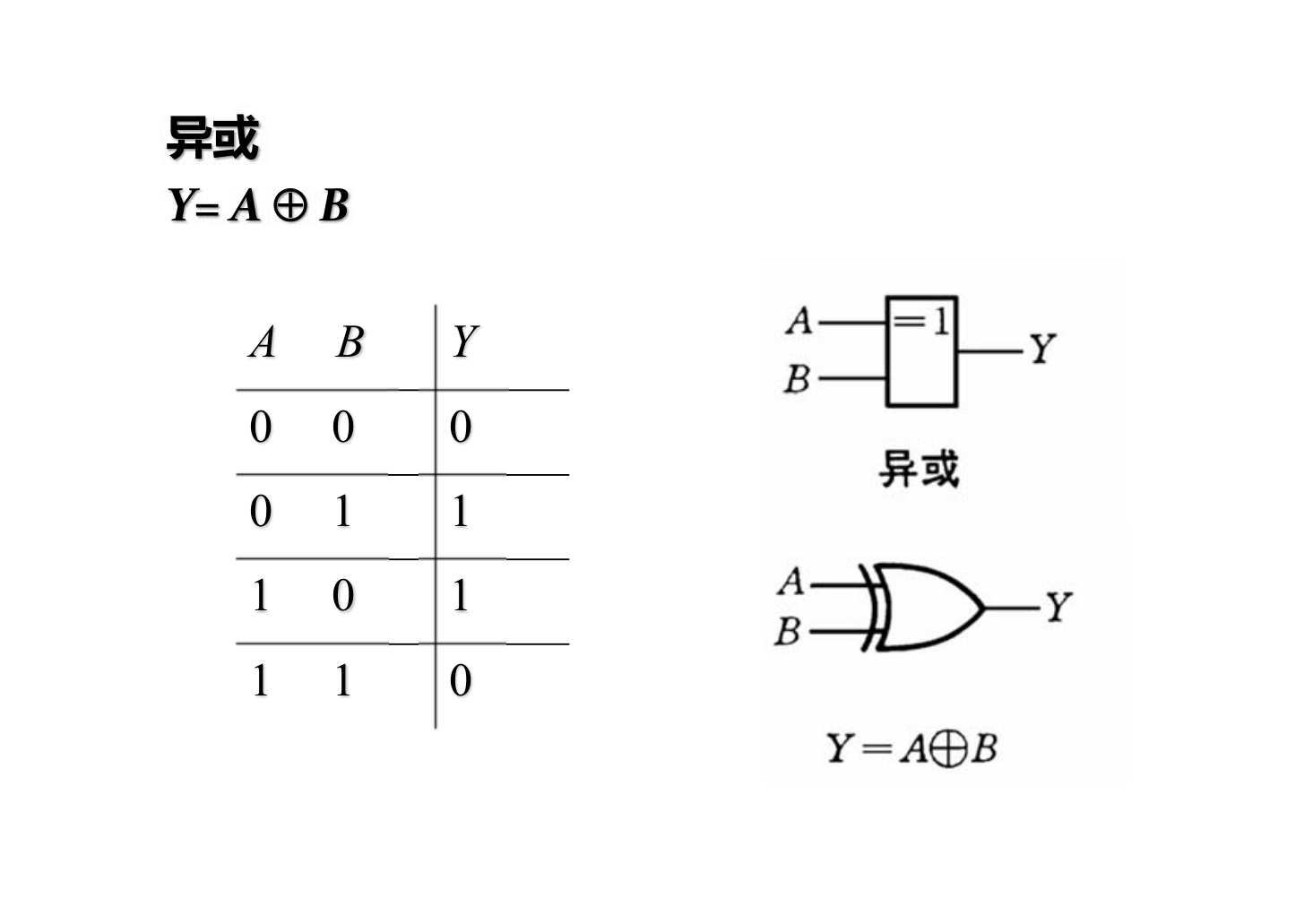### 逻辑运算基本公式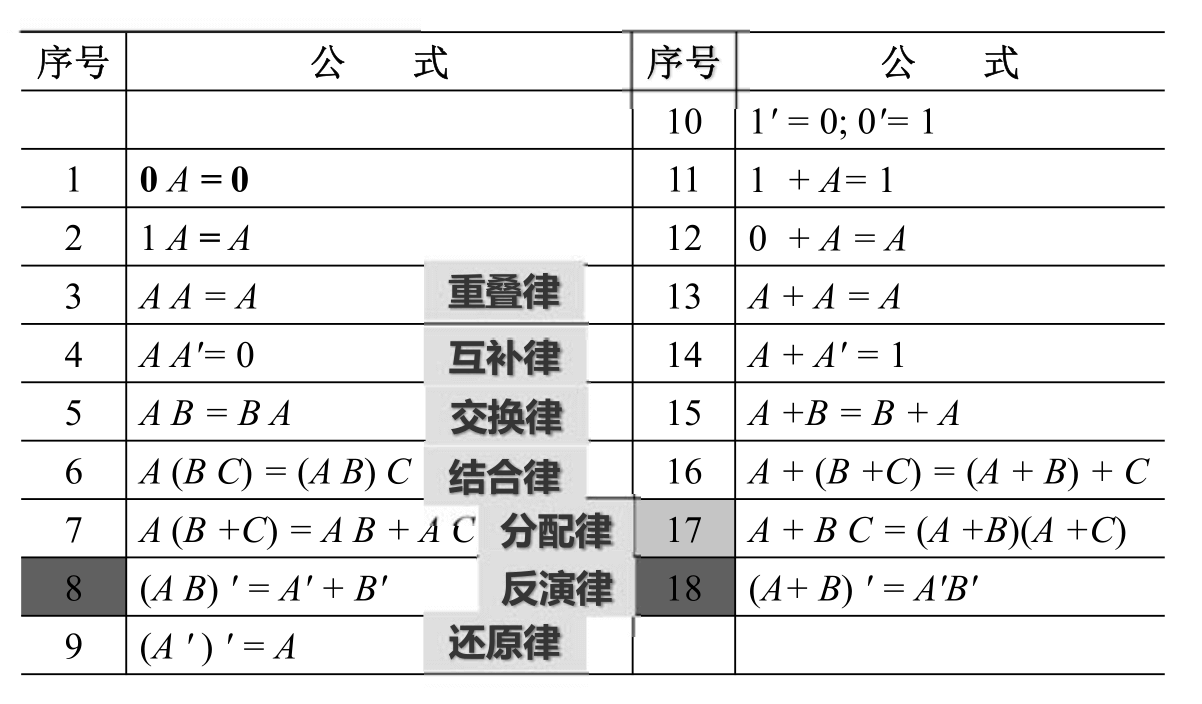$$(A B) ' = A' + B'$$
$$(A+ B)' = A'B'$$
$$A + B C = (A +B)(A +C)$$

### 逻辑运算常用公式### 逻辑代数基本定理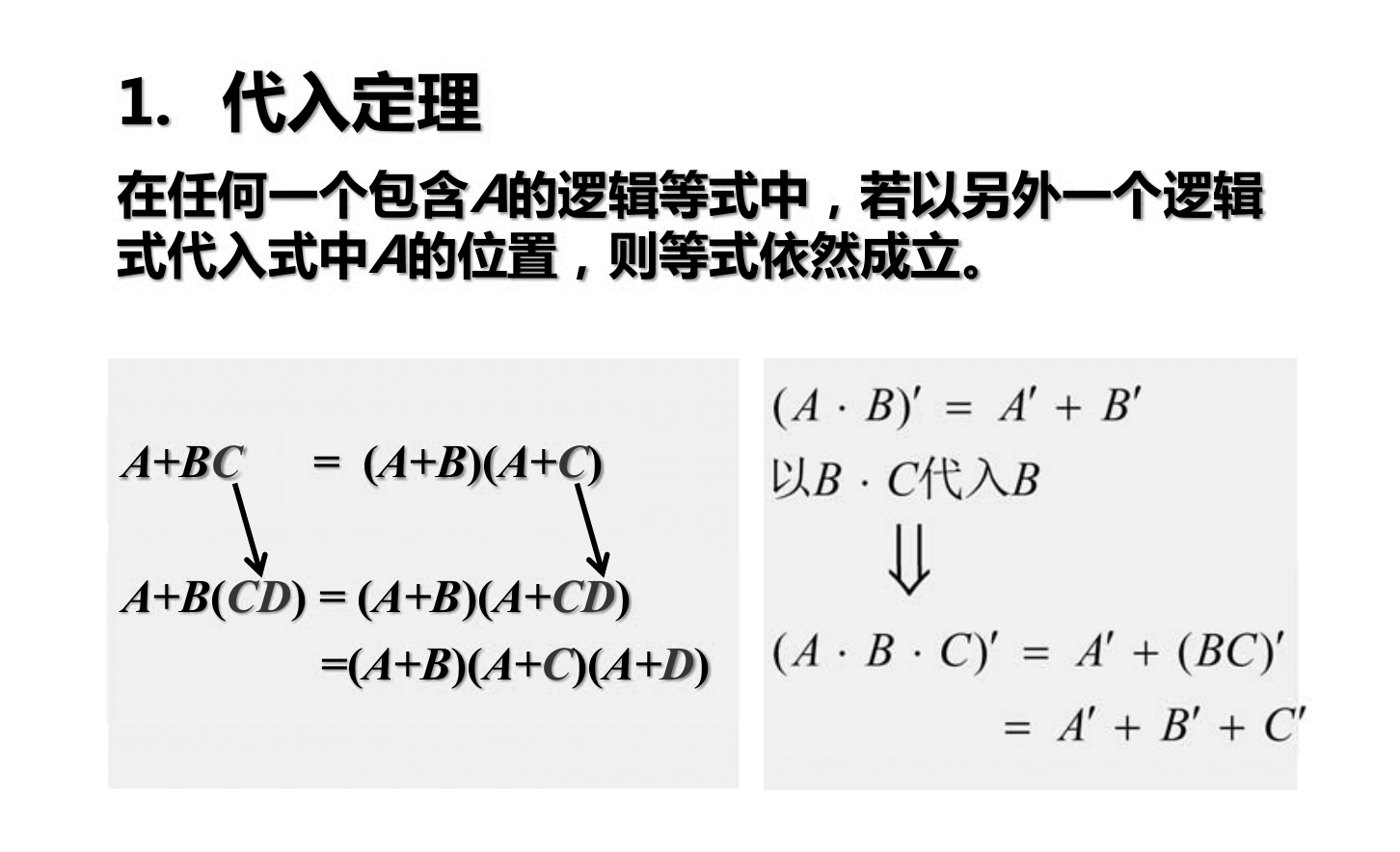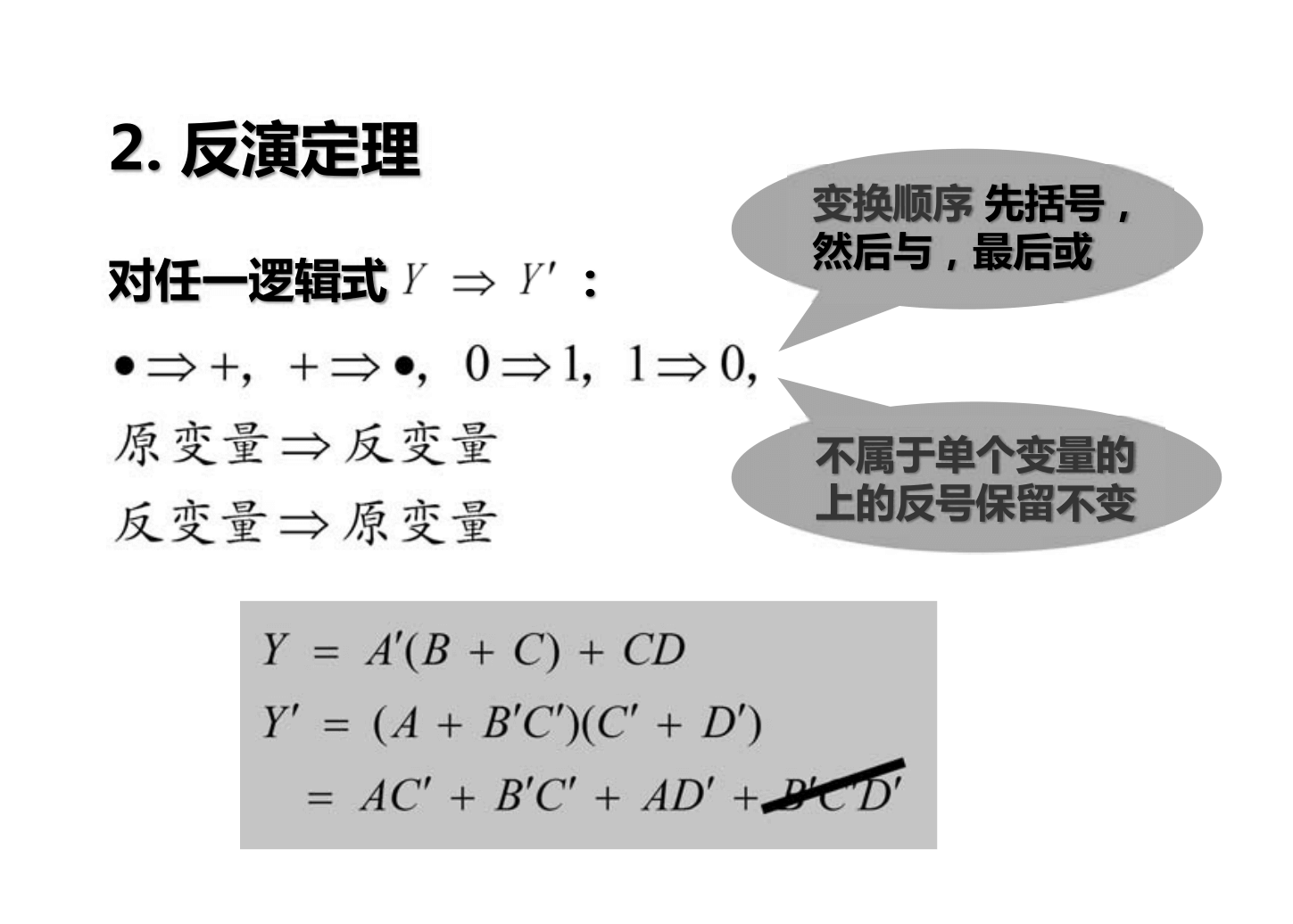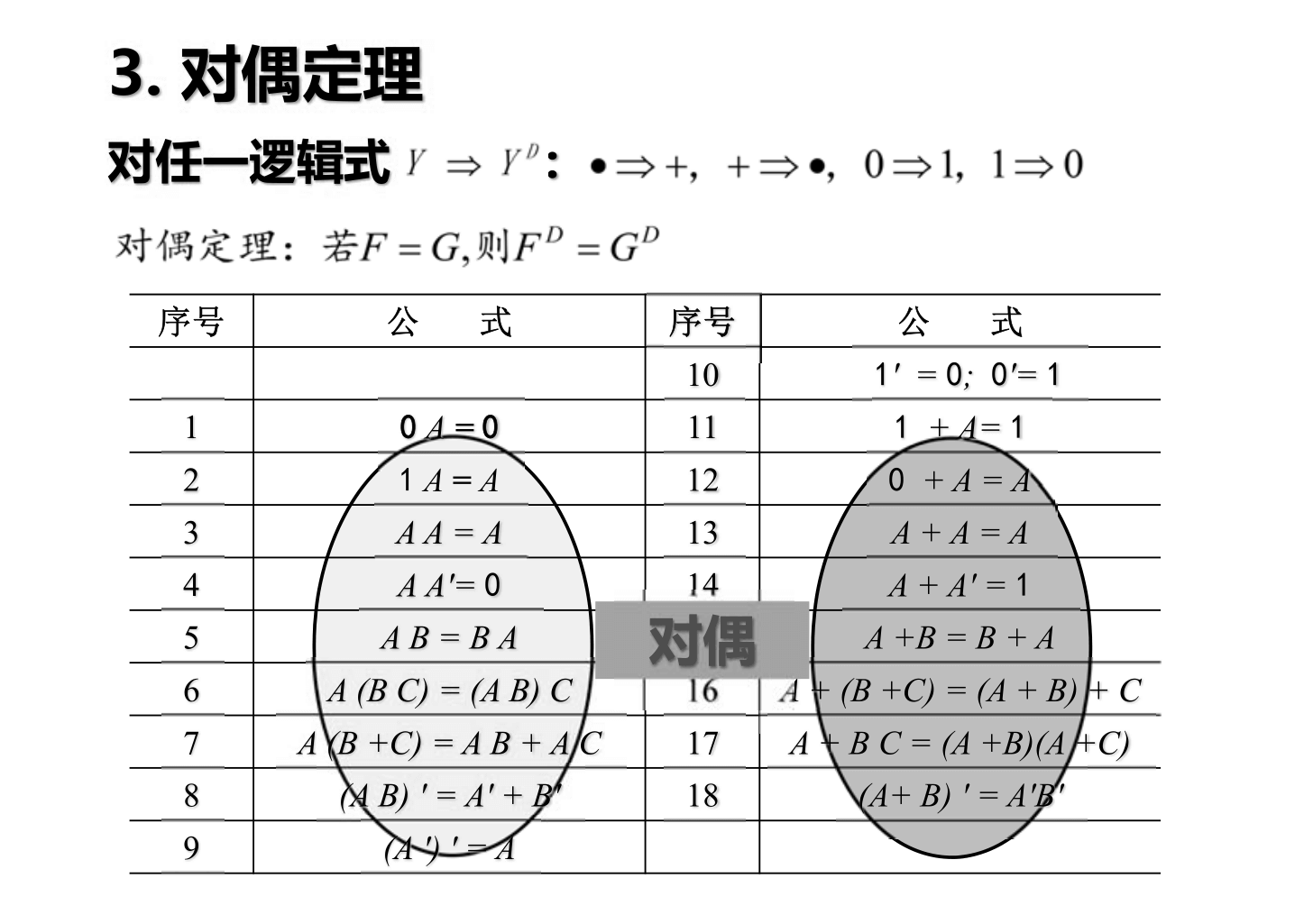### 逻辑函数的表示方法

• 真值表
• 逻辑式
• 逻辑图
• 波形图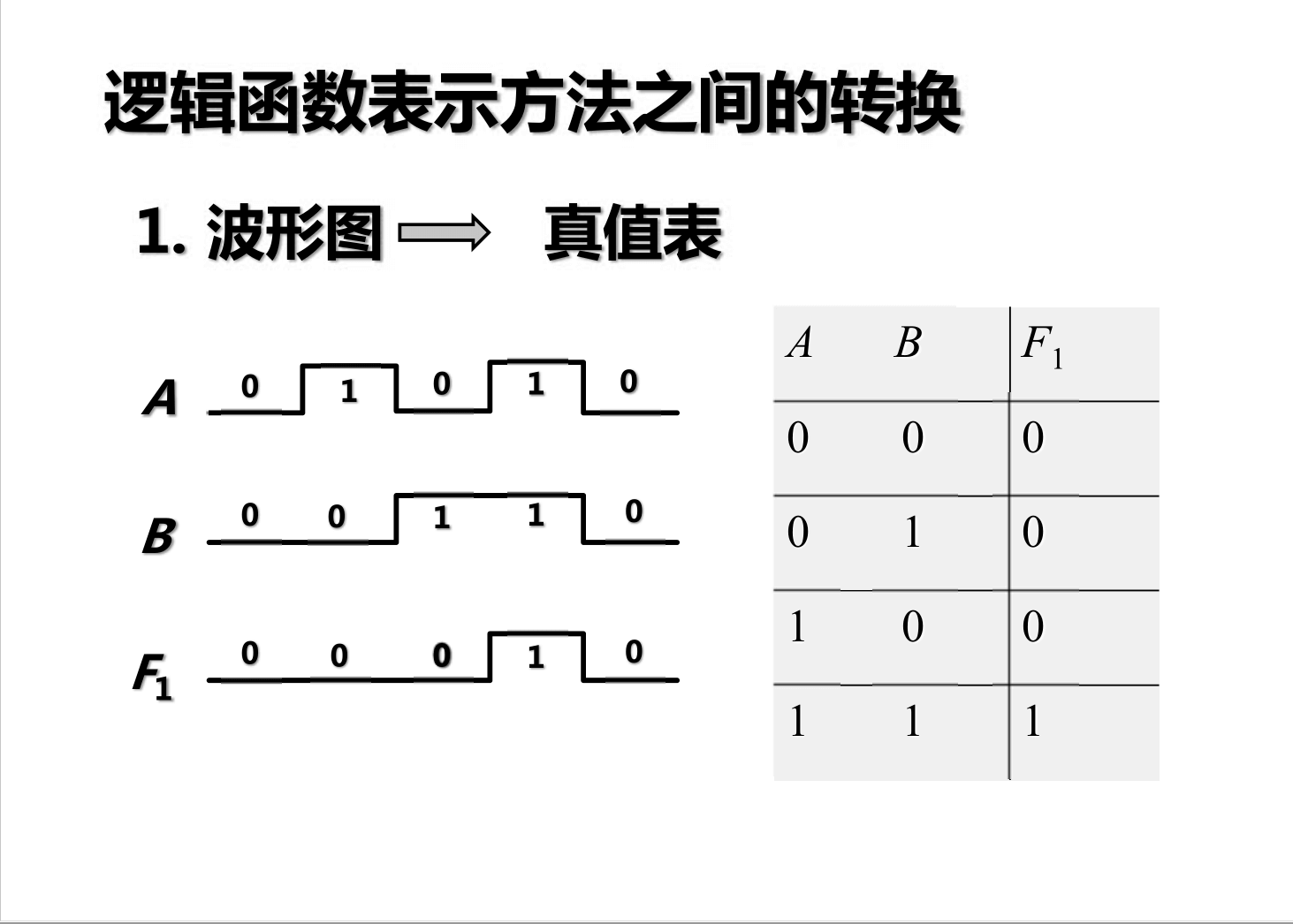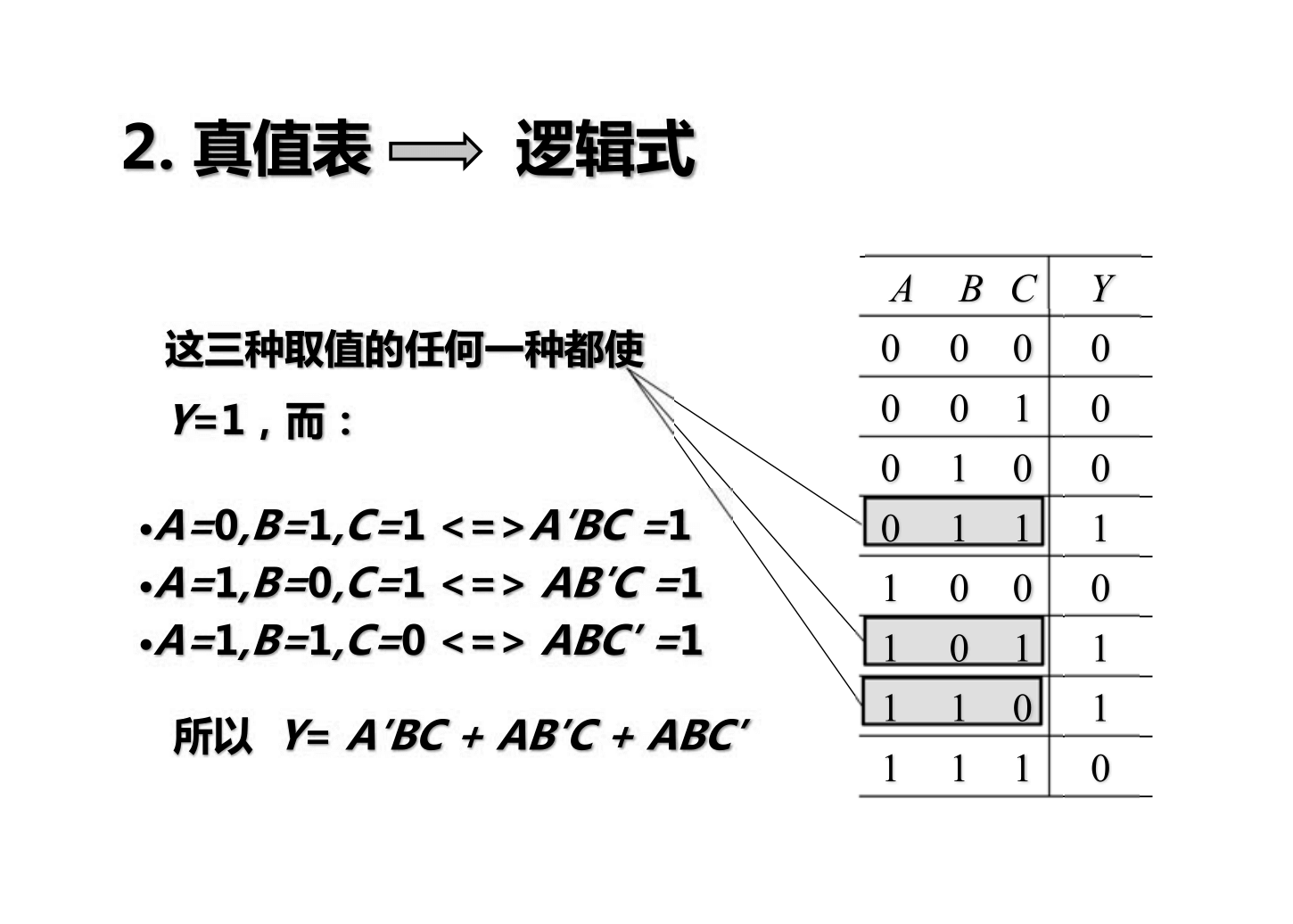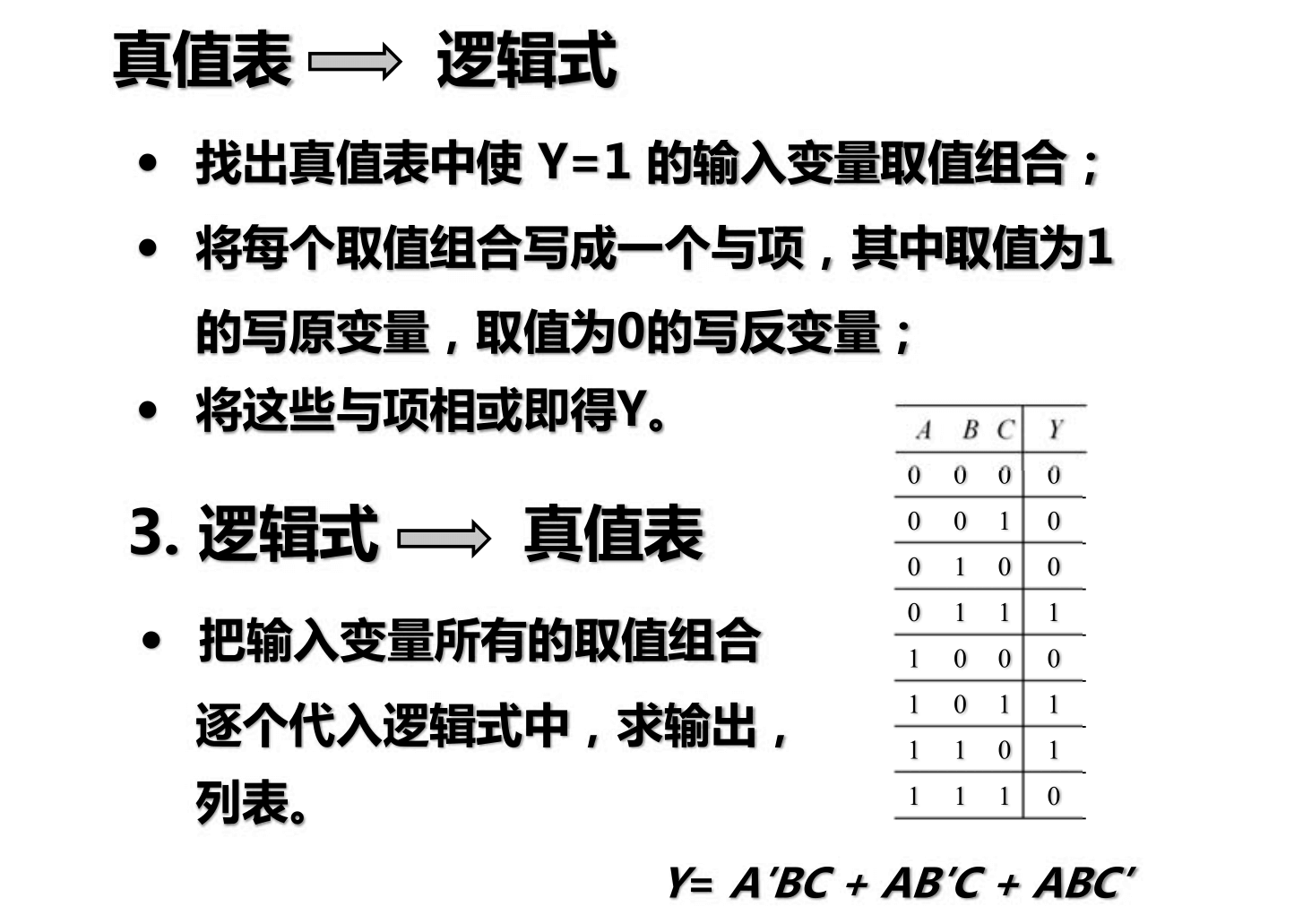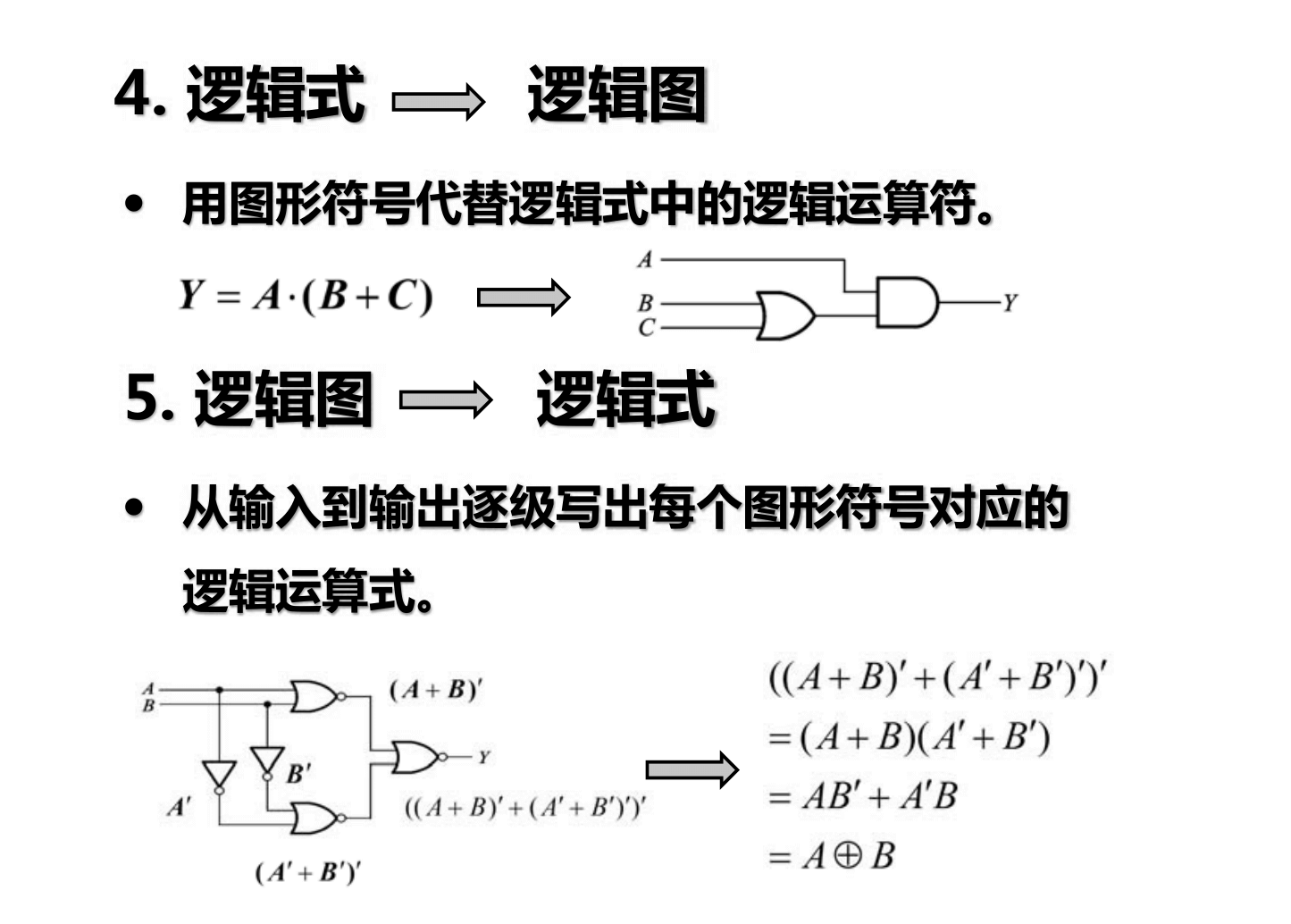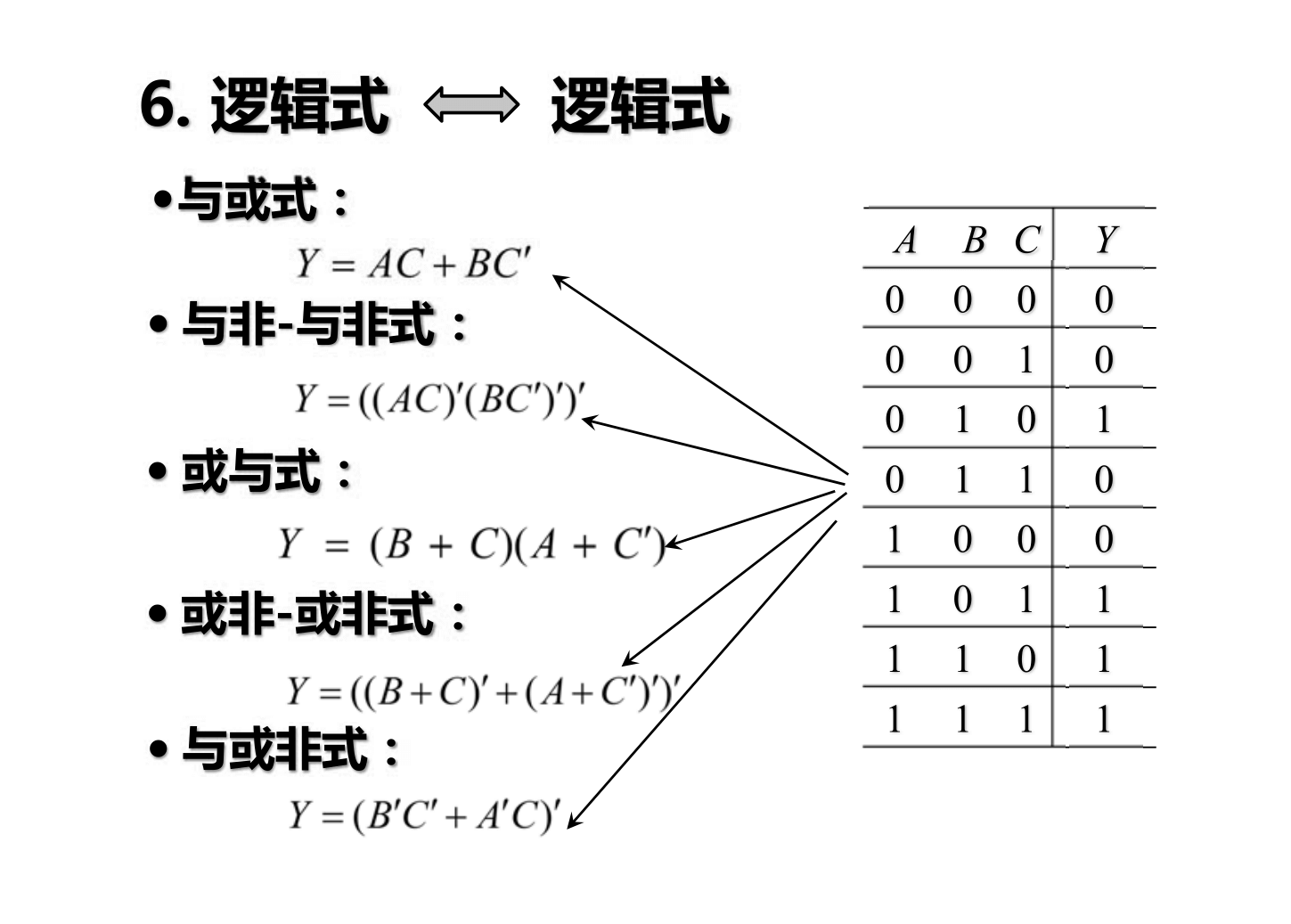### 逻辑函数的公式化简法

#### 卡诺图化简法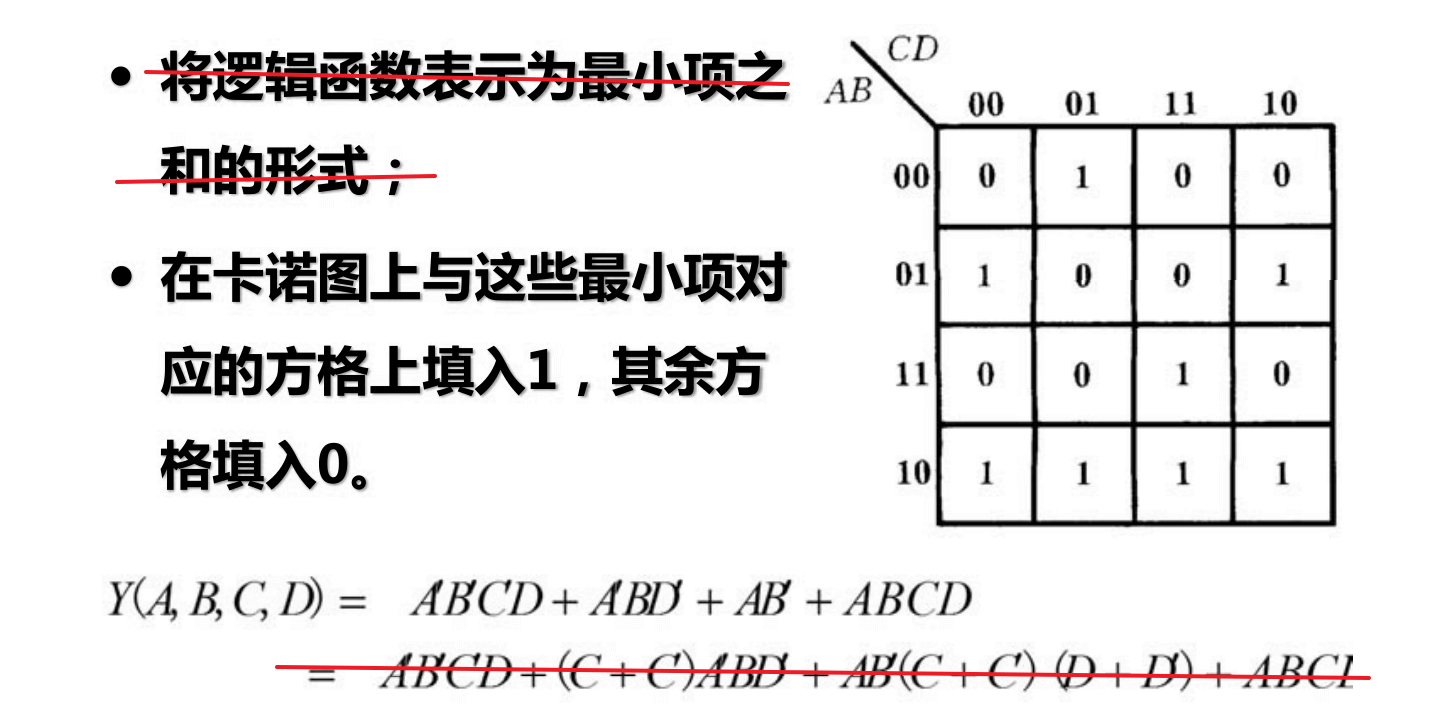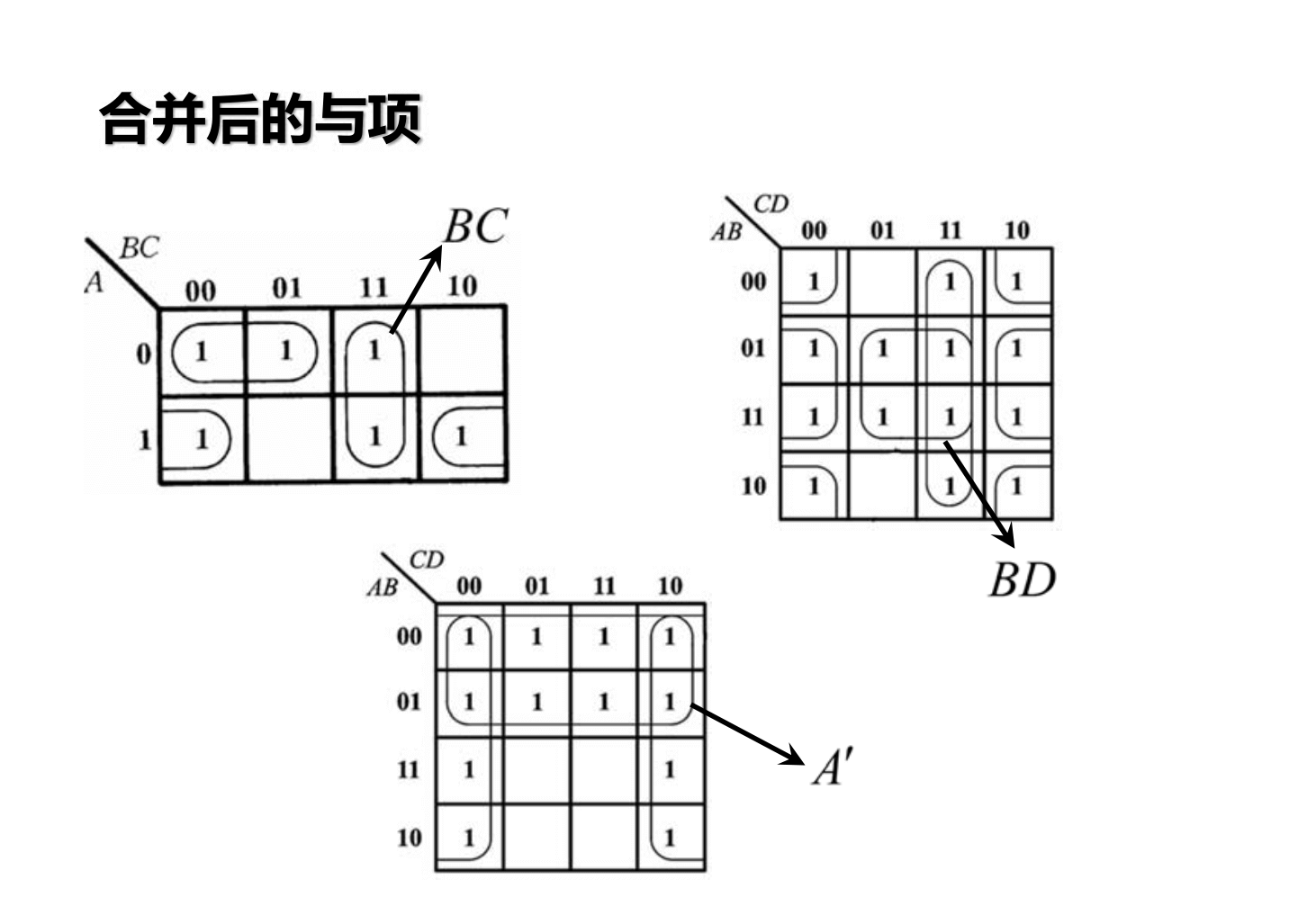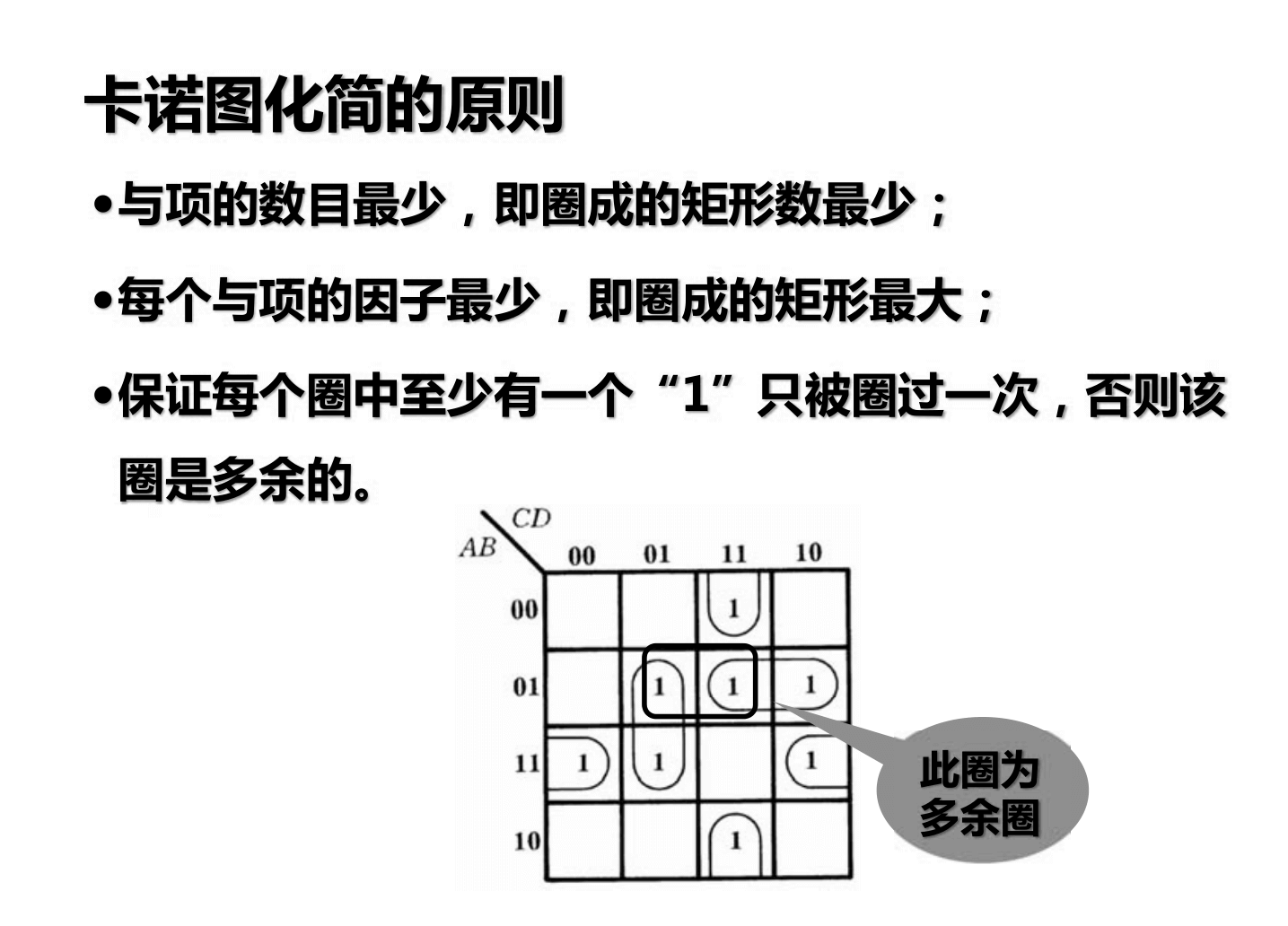## 门电路

### 正逻辑与负逻辑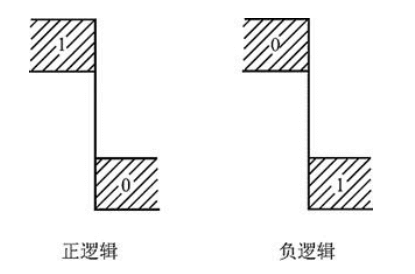### CMOS 管门电路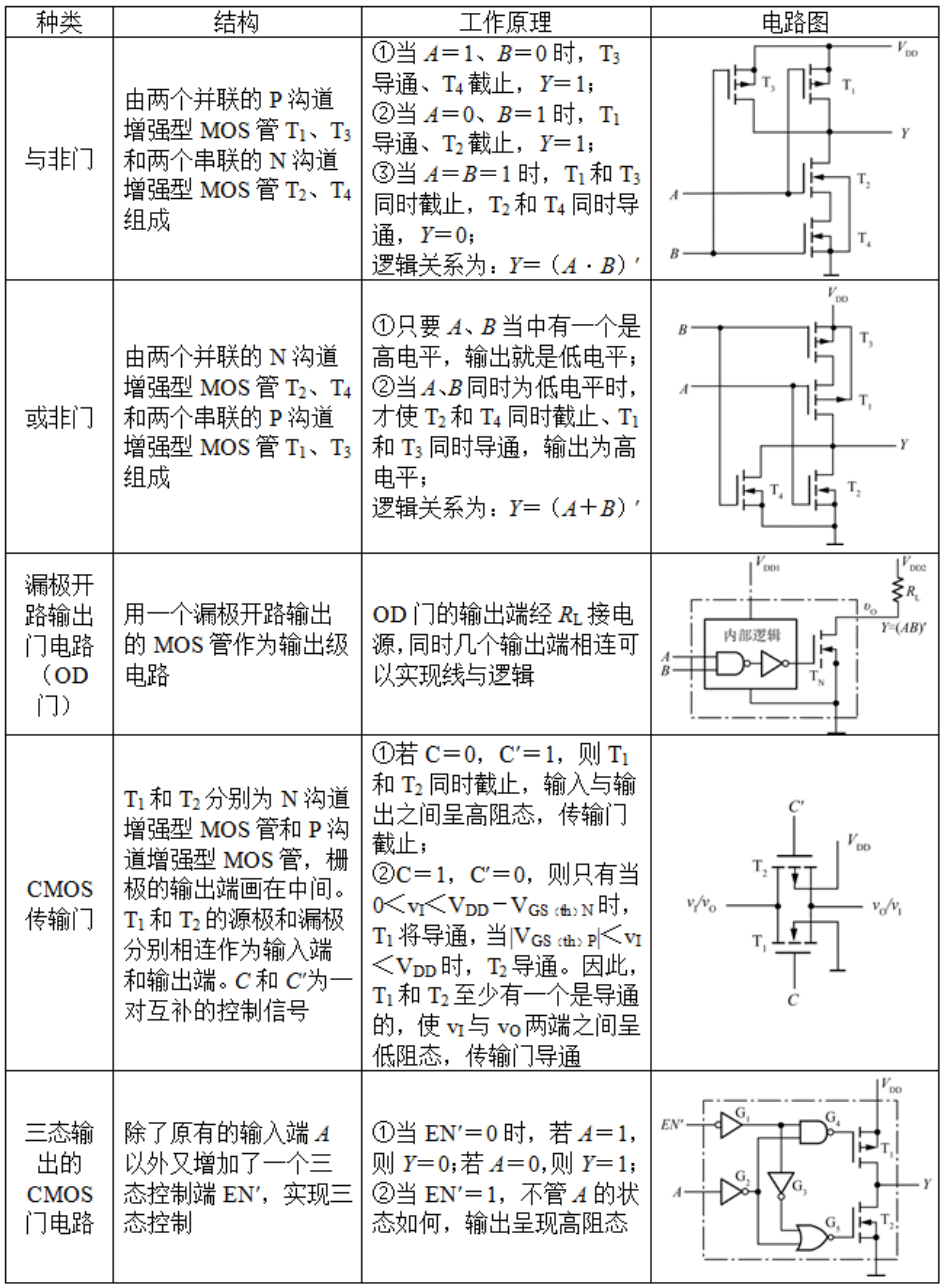## 参考与致谢

• 《编码：隐匿在计算机软硬件背后的语言》